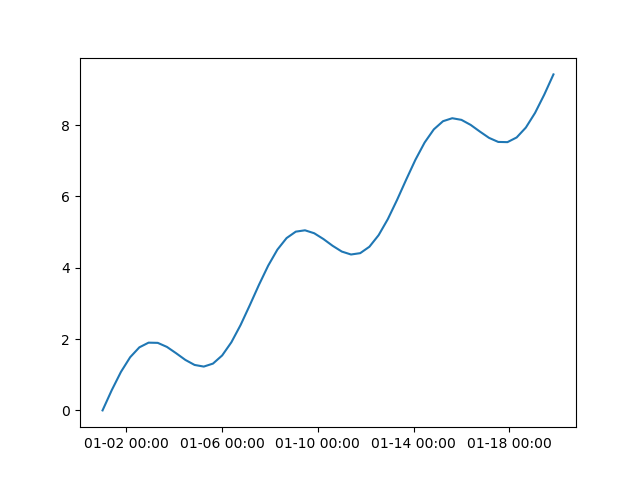# Examples

## Basic usage

nc-time-axis works by adding converters to matplotlib’s flexible units registry. To register its converters, simply import nc-time-axis. Then you will be able to make plots with `cftime.datetime` axes.

```In : import cftime

In : import matplotlib.pyplot as plt

In : import nc_time_axis

In : import numpy as np

In : fig, ax = plt.subplots(1, 1)

In : x = np.linspace(0, 6 * np.pi)

In : y = 0.5 * x + np.sin(x)

In : times = cftime.num2date(x, units="days since 2000-01-01", calendar="noleap")

In : ax.plot(times, y);

In : fig.show()
```## Setting the axes ticks and tick format

In the first example, the ticks and tick label formats were chosen automatically using heuristics in nc-time-axis. If you would like to explicitly set the tick positions and label format you may do so using `matplotlib.axes.Axes.set_xticks()` and `CFTimeFormatter`. The `CFTimeFormatter` takes in a date format string (see the Python documentation for acceptable format codes) and the calendar type of the axis (see the `cftime.datetime` documentation for valid calendar strings).

```In : fig, ax = plt.subplots(1, 1)

In : ax.plot(times, y);

In : ax.set_xticks([cftime.datetime(2000, 1, day, calendar="noleap") for day in range(2, 19, 4)]);

In : formatter = nc_time_axis.CFTimeFormatter("%m-%d %H:%M", "noleap")

In : ax.xaxis.set_major_formatter(formatter)

In : fig.show()
```